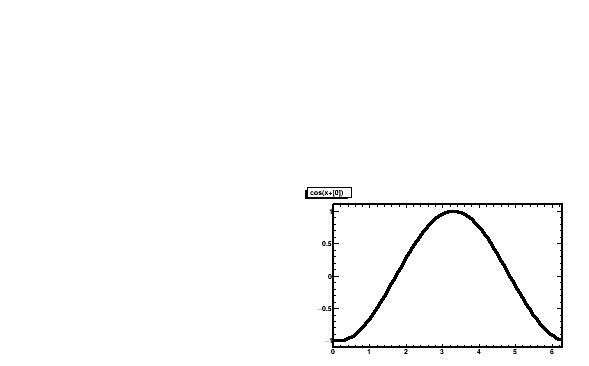# Multiple (similar) plots on Divided TCanvas

Hi all,
I am using PyROOT to plot mutiple (similar) histograms using TCanvas:: Divide():
I used TF1 for testing (see the following code).

``````import ROOT as r
N = 2
canvas = r.TCanvas("canvas object name","function",600,400)
canvas.Divide(N,N)

for i in range(N*N):
canvas.cd(i+1)
cosx = r.TF1('cosx{}'.format(i),'cos(x+)',0,6.284)
#     cosx = r.gDirectory.GetObject('cosx{}'.format(i))
cosx.SetParameter(0,i)
cosx.Draw()
canvas.Draw()
``````

the output look like this:I also tried the following (with no luck):

1. put `r.TF1(....)` outside the `for` loop, ending up with 4 identical plots.
2. uncomment the `gDirectory.Get` line, I got an error which says:
Template method resolution failed: Failed to instantiate “GetObject(std::string)”

I am sure that other users also have encounterred the problem (but I really cant find a precise way to describe the problem, so I decide to ask in the Newbie section). Could somebody suggest the best way to do this kind of plot for me ? Thanks in advance.

_ROOT Version: 6.24 (PyROOT via conda)
_Platform:Centos7
_Compiler: gcc9

Thanks. It worked !

Or you can use Draw(0 this way:

``````void junkai() {
int N = 2;
auto canvas = new TCanvas("canvas object name","function",600,400);
canvas->Divide(N,N);

for (int i=0; i<N*N; i++) {
canvas->cd(i+1);
auto cosx = new TF1(Form("cosx{%d}",i),"cos(x+)",0,6.284);
cosx->SetParameter(0,i);
cosx->Draw();
}
}
``````

thanks for the suggestion. It worked in macro but not in python. Is there other method than the DrawCopy() in PyROOT (just curious) ?

Btw, I also found that the object that is created in the `for` loop cant be accessed by running: `gDirectory->ls();`. Outside the loop, I have a TH3 object and (of course) the canvas. But only the TH3 was listed when `gDirectory->ls();` was run. Am I using the correct way to access the list of created Objects?

``````void PhaseMapTest(){

int NBinX = 18;
int NBinY = 9;
int NBinZ = 1000;

TH3F* phase_map = new TH3F("phase_map","phase map title;posiInitposX [mm]; posiInitPosY [mm]; posiInitTime [#mu s]",NBinX,-45,45,NBinY,-45,45,NBinZ,0,149.2);
phase_map->Sumw2();

int x = 8;
int y = 7;
phase_map->GetXaxis()->SetRange(x+1,x+1);

int N = 2;
auto canvas = new TCanvas("canvas object name","function",600,400);
canvas->Divide(N,N);

for (int i=0; i<N*N; i++) {
canvas->cd(i+1);
auto cosx = new TF1(Form("cosx{%d}",i),"cos(x+)",0,6.284);
cosx->SetParameter(0,i);
cosx->Draw();
};
canvas->Print("t.png");
gDirectory->ls();
``````

`gROOT->GetListOfCanvases()->ls();`
`gROOT->GetListOfFunctions()->ls();`

1 Like